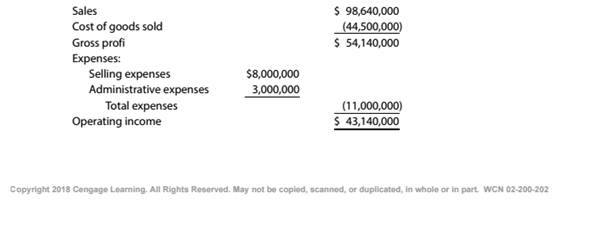Chapter 11, Problem 11.2.4P

Chapter
Section
Textbook Problem

Break-even sales under present and proposed conditions Kearney Company, operating at full capacity, sold 400,000 units at a price of $246.60 per unit during 20Y5. Its income statement for 20Y5 is as follows:The division of costs between fixed and variable is as follows:Management is considering a plant expansion program that will permit an increase of$8,631,000 (35.000 units at $246.60) in yearly sales. The expansion will increase fixed costs by$3,600,000 but will not affect the relationship between sales and variable costs.Instructions Compute the break-even sales (units) under the proposed program.

To determine

Concept Introduction:

Cost Volume Profit (CVP) Analysis:

The Cost Volume Profit analysis is the analysis of the relation between cost, volume, and profit of a product. It analyzes the cost and profits at the different level of production, in order to determine the breakeven point and required the level of sales to earn the desired profit.

Contribution margin means the margin that is left with the company after recovering variable cost out of revenue earned by selling smart phones. The formula:

Contribution margin = Sales - Variable cost.

Similarly contribution margin ratio = Contribution/sales

Breakeven Point:

The Breakeven point is the level of sales at which the net profit is nil. It can be explained as a situation where the business is generating a sale that is equal to the expenses incurred and hence no profits no loss. Breakeven point in units is calculated with the help of following formula:

Breakeven point (units) = Total Fixed Costs(Sales Price Per unit -Variable Cost per unit)

To Calculate:

The Breakeven unit sales under the proposed program

Explanation

The Breakeven unit sale under the proposed program is calculated as follows:

 Total Fixed Cost (A) (16860000+3600000) \$ 20,460,000

Still sussing out bartleby?

Check out a sample textbook solution.

See a sample solution

The Solution to Your Study Problems

Bartleby provides explanations to thousands of textbook problems written by our experts, many with advanced degrees!

Get Started

Identify the five major column headings on a work sheet.

College Accounting, Chapters 1-27 (New in Accounting from Heintz and Parry)

REQUIRED LUMP SUM PAYMENT Starting next year, you will need 10,000 annually for 4 years to complete your educat...

Fundamentals of Financial Management, Concise Edition (with Thomson ONE - Business School Edition, 1 term (6 months) Printed Access Card) (MindTap Course List)

Explain how safety stock is used to deal with demand uncertainty.

Cornerstones of Cost Management (Cornerstones Series)